• Shuffle
Toggle On
Toggle Off
• Alphabetize
Toggle On
Toggle Off
• Front First
Toggle On
Toggle Off
• Both Sides
Toggle On
Toggle Off
Toggle On
Toggle Off
Front

## Card Range To Study

throughPlay buttonPlay buttonProgress

1/32

Click to flip

Use LEFT and RIGHT arrow keys to navigate between flashcards;

Use UP and DOWN arrow keys to flip the card;

H to show hint;

 Coulomb’s Law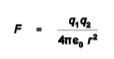q= electrical point chargesr=distance**FORCE IS STRONGLY EFFECTS BY DISTANCE. One coulomb is N0 /F charges where N0 is Avogadro’s number and F is the Faraday: 6.02 x 1023 charges mol-1 / 96,485 coulombs mol-1 = 6.24 x 10^18 elementary charges. The charge on the electron = 1.6 x 10^-19 coulomb. potential energy = U We define the potential at any place A in space as being the work to bring a positive unit charge from infinite separation to point A. Because it takes work to bring a positive charge towards a positive fixed charge, the potential surrounding a positive charge is positive Conversely, since a positive test charge is accelerated towards a negative fixed charge, the potential around a negative charge is negative. Separation of charge produces a potential proportional to the charge. The potential around a positive charge is positive; the potential around a negative charge is negative. True Ohm’s Law relates current to potential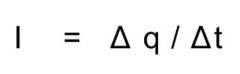I is given in amperes = coulomb/sI= Q/ driving force 1/resistance = Q (conductance) Current is defined as the movement of positive charge so that movement of cations (positive ions) constitutes a current in the direction of the flow; movement of anions (negative ions) makes a current in the opposite direction to the flow. The current due to an ion is related to its flow by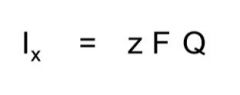where current (I) is in coulombs s-1, flow (Q) is in mol s-1 and zF converts mol to coulombs. The sign of z shows that current is in the same direction as flow for cations, opposite for anions. conductors materials that offer little resistance insulators offer high resistance- examples are membrane lipids and the myelin sheath that surrounds nerve axons ohms law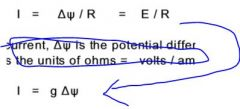where I is the current, (fork thingy) is the potential difference, often symbolized as E or V, and R is the resistance. where g = 1 / R is the conductance. The SI unit for conductance is the siemen = amp / volt for an axon interior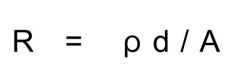d is the distance or length, A is the cross-sectional area and p is the specific resistance of the electrolyte fluid in the axon interior. R has the units of ohm resistance of a patch of membrane R(m) / AreaRm= specific resistance dielectric insulating material between 2 plates capacitive current- there is no flow across the dielectric but there is a flow in the circuit The flow of positive ions onto the top plate of the capacitor produces an electric field (a force per unit charge) that repels positive charges from the bottom plate. This movement of charges away from the bottom plate is the capacitive current. As the charges move away, there is a separation of charges on the capacitor and it now has a potential difference. Charges continue to move until the potential across the capacitor is equal but opposite to the potential across the battery Capictance *KNOW THIS!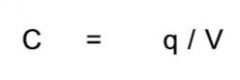C is the capacitance, q is the charge separated and V is the voltage across the capacitor The ability to store charge depends on the physical characteristics of the capacitorKNOW THE RLTSP between AREA and DISTANCE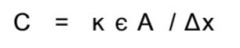k= dielectric constantx= distance that seperates platesE= electrical permittivity t=RCt= timeRC= TIME CONSTANT because it describes the time taken to charge the capacitor If t = RC, then q = qinfitnity (1 - 1/e)q= the full charge (EC)1/e = .37 ions are subjected to two kinds of forces concentration gradient potential gradient* The forces due to these gradients exactly balance each other at the equilibrium potential given by the Nernst equation* T=RC defines how fast the capicator is charging charge=.63current = .37 ? equilibrium potential is reached when the system is at equilibrium ∆G=0 true NERST equation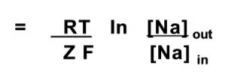For a solute with z=+1 and conversting ln to log, the Nerst equation is often written as If z is negative, like CL-, then it would be -61.5log...!!(when volts is in mV)***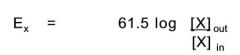The equilibrium potentials are those at which flux is zero for the indicated ion. True The resting potential is given by the Goldman-Hodgkin-Katz equation, also called the constant field equation because its derivation assumes that the electric field in the membrane is constant.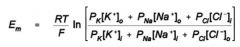Here Px is the permeability of ion X. Note that in this equation [K+]o and [Na+]o appear in the numerator, while [Cl-]I appears there. This is a consequence of their opposite signs of electric charge. The current carried by an ion is directly proportional to the flow of the ion true If the concentration of the ion is higher, there is a higher flow of ions, a greater current, in response to a potential difference than if the concentration is lower. True As Em moves away from the equilibrium potential, the current can be described as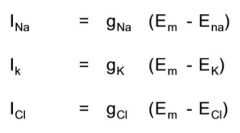gNa and gK are the chord conductances for the respective ions. ENa and EK are the equilibrium potentials. Em is the membrane potential. chord conductance slope of the chord connecting the curve to its reversal potential. The chord conductance is the slope of the line connecting the GHK current to its equilibrium potential.THEY ARE ALWAYS POSITIVE At rest the total current across the membrane is zero. It doesnt mean each current is 0, but they sum up to 0. Itotal = 0 If it were not, then the membrane would be building up charge or discharging and the membrane potential would not be stable - it would not be the resting condition. chord conductance equation. It basically says the resting membrane potential is the chord conductance weighted average of the equilibrium potentials for each of the ions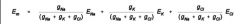There is some small leak of ions, K+ out of the cell and Na+ into the cell, during rest. These leaks are kept in check by the Na-K-ATPase that pumps K+ back into the cell and Na+ out of the cell. This pump is electrogenic ( it carries a current because the stoichiometry of K+ is 2 and Na+ is 3.) Thus it carries an outward current that polarizes the cell. At rest this makes a small contribution: inhibition of the pump by ouabain depolarizes the cell by about 2mV.